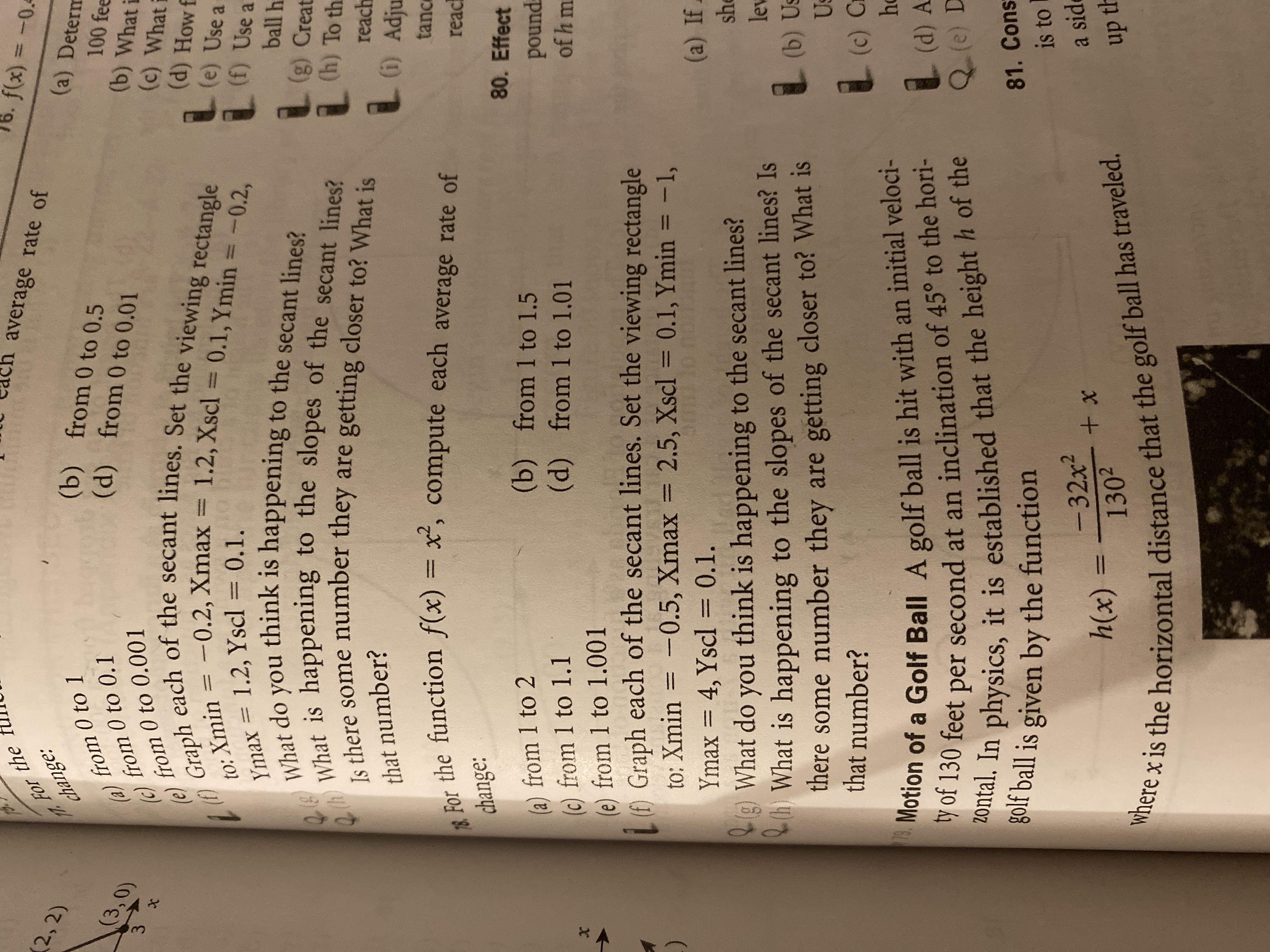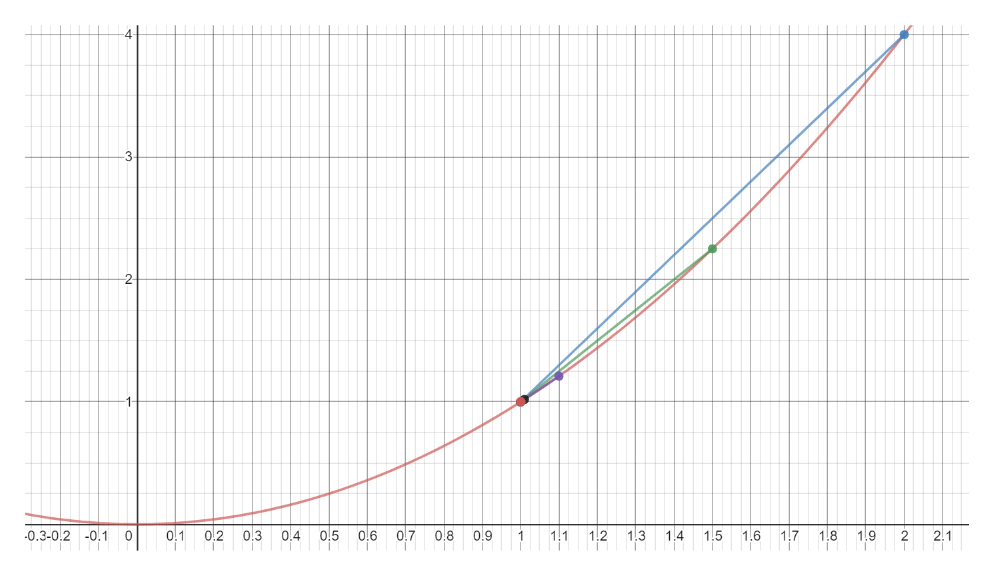# f(x)77 FOr thechange:average rate of=-0.42,2)(a) Determ(b) from 0 to 0.5(d) from 0 to 0.01a)from 0 to 10 to 0.1100 fee(b) What i(c) What(d) How f(e) Use a(f) Use a(3,0)(c)from 0 to 0.001from3Graph each of the secant lines. Set the viewing rectanglele)to: Xmin =-0.2, Xmax = 1.2, Xscl = 0.1, Ymin =Ymax = 1.2, Yscl = 0,1.What do you think is happening to the secant lines?-0.2,ball h(g) Creat(h) To th@thWhat is happening to the slopes of the secant lines?Is there some number they are getting closer to? What isreachAdjuthat number?tanc78 For the function f(x) = x', compute each average rate ofreacchange:80. Effect(b) from 1 to 1.5(d) from 1 to 1.01(a) from 1 to 2(c) from 1 to 1.1(e) from 1 to 1.001Graph each of the secant lines. Set the viewing rectangleto: Xmin = -0.5, Xmax = 2.5, Xscl = 0.1, Ymin = -1,Ymax = 4, Yscl 0.1gWhat do you think is happening to the secant lines?What is happening to the slopes of the secant lines? Isthere some number they are getting closer to? What isthat number?poundof h mX(a) Ifshlev(b) UsUs() C78Motion of a Golf Ball A golf ball is hit with an initial veloci-ty of 130 feet per second at an inclination of 45° to the hori-Zontal. In physics, it is established that the height h of thegolf ball is given by the functionho(d) AQ(e) D81. Consis to-32x2+ xa sidh(x)1302up thwhere x is the horizontal distance that the golf ball has traveled.

Question
109 views

Hello I need some help with 78-f,g and hhelp_outlineImage Transcriptionclosef(x) 77 FOr the change: average rate of =-0.4 2,2) (a) Determ (b) from 0 to 0.5 (d) from 0 to 0.01 a)from 0 to 1 0 to 0.1 100 fee (b) What i (c) What (d) How f (e) Use a (f) Use a (3,0) (c) from 0 to 0.001 from 3 Graph each of the secant lines. Set the viewing rectangle le) to: Xmin =-0.2, Xmax = 1.2, Xscl = 0.1, Ymin = Ymax = 1.2, Yscl = 0,1. What do you think is happening to the secant lines? -0.2, ball h (g) Creat (h) To th @thWhat is happening to the slopes of the secant lines? Is there some number they are getting closer to? What is reach Adju that number? tanc 78 For the function f(x) = x', compute each average rate of reac change: 80. Effect (b) from 1 to 1.5 (d) from 1 to 1.01 (a) from 1 to 2 (c) from 1 to 1.1 (e) from 1 to 1.001 Graph each of the secant lines. Set the viewing rectangle to: Xmin = -0.5, Xmax = 2.5, Xscl = 0.1, Ymin = -1, Ymax = 4, Yscl 0.1 gWhat do you think is happening to the secant lines? What is happening to the slopes of the secant lines? Is there some number they are getting closer to? What is that number? pound of h m X (a) If sh lev (b) Us Us () C 78Motion of a Golf Ball A golf ball is hit with an initial veloci- ty of 130 feet per second at an inclination of 45° to the hori- Zontal. In physics, it is established that the height h of the golf ball is given by the function ho (d) A Q(e) D 81. Cons is to -32x2 + x a sid h(x) 1302 up th where x is the horizontal distance that the golf ball has traveled. fullscreen
check_circle

Step 1

f) Graphed the function and graphed the secant lines.help_outlineImage Transcriptionclose01 0.2 1.5 1.6 1.7 0.3-0.2 -0.1 O 0.3 0.4 0.5 0.6 0.7 0.8 12 13 1.8 19 0.9 1.1 2 2.1 1 1.4 fullscreen
Step 2

g) Answer: Slope of the secant lines are decreasing and they are getti...

### Want to see the full answer?

See Solution

#### Want to see this answer and more?

Solutions are written by subject experts who are available 24/7. Questions are typically answered within 1 hour.*

See Solution
*Response times may vary by subject and question.
Tagged in

### Other